Monday 13th July 2020

CBSE Guess > Papers > Question Papers > Class XII > 2004 > Maths > Outside Delhi Set-III

MATHS (Set III—Outside Delhi)

Except for the following questions, all the remaining questions have been asked in Set I and Set II.

SECTION - A

Q. 1. Using properties of determinants, Prove that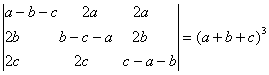Q. 4. Solve the differentia equation: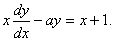Q. 9. Evaluate: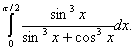Q. 13. Evaluate: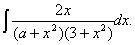Or

Evaluate: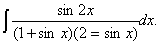Q. 15. Differentiate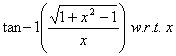Q. 18. Find the area of the region bounded by the curve x2 = 4y and the line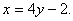SECTION - B

Q. 19. Prove that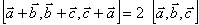Or

Use vector to prove the following:
If a, b and are the lengths of the sides opposite respectively to angles A, b and C of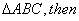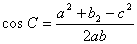Q. 20. Show that the area of a parallelogram having diagonals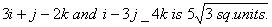Q. 21. Show that the line whose vector equation is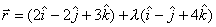is parallel to the plane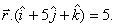Also find the distance between them.

Q. 22. Find the Cartesian and vector equations of a which passes through the point (1, 2, 3) and is parallel to the line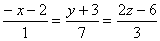Q. 23. Two forces of 8N and 6N act at a point and are inclined to each other at an angle of 600. Find the magnitude and direction of the resultant.

SECTION - C

Q. 21. A factory has three machines A, B and C, which produce 100, 200 and 300 items of a particular type daily. The machines produce 2% 3% and 5% defective items respectively. One day when the production was over, an item was pocked up randomly and it was fond to defective. Find the probability that it was produced by machine A.

Q. 25. A radio manufacturer produce x sets per week and sells at Rs. P per set, where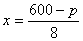. The cost of production of x sets is Rs. (x2 +78x+2000). Find the number of sets to be produced and sold per week so as to have maximum profit. Calculate the maximum profit also.

 Maths 2004 Question Papers Class XII Delhi Outside Delhi Compartment Delhi Compartment Outside DelhiSet ISet ISet ISet ISet IISet IISet IISet IISet IIICBSE 2004 Question Papers Class XII